## Morgan-Voyce Polynomial

Polynomials related to the Brahmagupta Polynomials. They are defined by the Recurrence Relations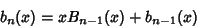(1)(2)

for, with(3)

Alternative recurrences are(4)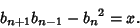(5)

The polynomials can be given explicitly by the sums(6)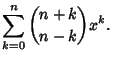(7)

Defining the Matrix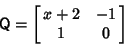(8)

gives the identities(9)(10)

Defining(11)(12)

gives(13)(14)

and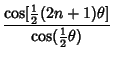(15)(16)

The Morgan-Voyce polynomials are related to the Fibonacci Polynomialsby(17)(18)

(Swamy 1968).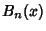satisfies the Ordinary Differential Equation(19)

andthe equation(20)

These and several other identities involving derivatives and integrals of the polynomials are given by Swamy (1968).

References

Lahr, J. Fibonacci and Lucas Numbers and the Morgan-Voyce Polynomials in Ladder Networks and in Electric Line Theory.'' In Fibonacci Numbers and Their Applications (Ed. G. E. Bergum, A. N. Philippou, and A. F. Horadam). Dordrecht, Netherlands: Reidel, 1986.

Morgan-Voyce, A. M. Ladder Network Analysis Using Fibonacci Numbers.'' IRE Trans. Circuit Th. CT-6, 321-322, Sep. 1959.

Swamy, M. N. S. Properties of the Polynomials Defined by Morgan-Voyce.'' Fib. Quart. 4, 73-81, 1966.

Swamy, M. N. S. More Fibonacci Identities.'' Fib. Quart. 4, 369-372, 1966.

Swamy, M. N. S. Further Properties of Morgan-Voyce Polynomials.'' Fib. Quart. 6, 167-175, 1968.